# Rectangle

The rectangle area is 182 dm2, its base is 14 dm. How long is the other side? Calculate its perimeter.

b =  13 dm
o =  54 dm

### Step-by-step explanation: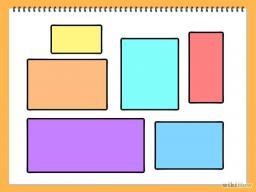Did you find an error or inaccuracy? Feel free to write us. Thank you!#### You need to know the following knowledge to solve this word math problem:

We encourage you to watch this tutorial video on this math problem:

## Related math problems and questions:

• Parallelogram - sideCalculate the length of the side of a parallelogram whose area is 175 dm2 and height to that side is 24.2 dm long.
• Area of rectangleCalculate the rectangle area in square meters whose sides have dimensions a = 80dm and b = 160dm.
• Two walls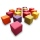Calculate the surface area of a cube in m2 if you know that the area of its two walls is 72 dm2.
• Folding tableThe folding kitchen table has a rectangular shape with an area of 168dm2 (side and is 14 dm long). If necessary, it can be enlarged by sliding two semi-circular plates (at sides b). How much percent will the table area increase? The result round to one-hu
• Square plate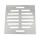The locksmith cut a square metal plate with a sidelong 6 dm into two identical rectangular openings with 2.5 dm and 2 dm dimensions. Calculate the remainder of the square plate.
• The parallelogram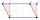The parallelogram has one side 2 dm long, which is one-sixth of its circumference. How many cm does the other side of the parallelogram measure?
• Edge of prismThe regular quadrilateral prism has a surface of 250 dm2, its shell has a content of 200 dm2. Calculate its leading edge.
• SquaresA square of 12 dm perimeter is cut smaller square, whose side is the third side of the square. What is the area of the rest?
• Bathroom 2A bathroom is 2.4 meters long and 1.8 meters wide. How many square tiles 1 dm on each side are to be used to cover it?
• Area of squareCalculate the content area of the square whose perimeter is 24 dm.
• Four prismsQuestion No. 1: The prism has the dimensions a = 2.5 cm, b = 100 mm, c = 12 cm. What is its volume? a) 3000 cm2 b) 300 cm2 c) 3000 cm3 d) 300 cm3 Question No.2: The prism base is a rhombus with a side length of 30 cm and a height of 27 cm. The height of t
• Octagon from rectangleFrom tablecloth rectangular shape with dimensions of 4 dm and 8 dm we cuts down the corners in the shape of isosceles triangles. It thus formed an octagon with area 26 dm2. How many dm2 we cuts down?
• Playground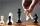On the special playground, there are 81 square sectors, each with a side of 5 m. How many players can fit on the playground if each player needs a 75 m2 area to play?
• ShapePlane shape has a maximum area 677 mm2. Calculate its perimeter if perimeter is the smallest possible.
• Perimeter of the circleCalculate the perimeter of the circle in dm, whose radius equals the side of the square containing 0.49 dm2?
• Rectangle A2dim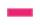Calculate the side of the rectangle, if you know that its area is of 2590 m2 and one side is 74 m.
• Two sides paintThe door has the shape of a rectangle with dimensions of 260cm and 170cm. How many cans of paint will be needed to paint this door if one can of paint cover 2m2 of the area? We paint the doors on both sides.